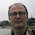## Pages

### Using recursive_directory_iterator

Let's modify the code we have just written to list a directory tree getting rid of the recursion. To do the trick we use a relatively new Boost Filesystem feature, the recursive_directory_iterator, an iterator that runs on all the files in the current directory traversing recursively all its subdirectories.

Warning! Boost 1.56 introduced a behavior change that makes the code showed here subtly wrong. Please, check the code provided by LukeM for a working patch. Thank you Luke!

In this way we plan to simplify our code, letting to the iterator the task of translating the (potentially very complex) tree structure in a flat one, and we keep for ourselves the easier task of iterating on each element.

To be honest, in such a simple example as this one, you could have the impression that we are increasing the code complexity instead. But in a real case the balance should be different.

Creating a recursive_directory_iterator

We are using a different iterator, so we change accordingly the class that creates it:
```boost::filesystem::recursive_directory_iterator createRIterator(boost::filesystem::path path)
{
try
{
return boost::filesystem::recursive_directory_iterator(path);
}
catch(boost::filesystem::filesystem_error& fex)
{
std::cout << fex.what() << std::endl;
return boost::filesystem::recursive_directory_iterator();
}
}
```
Dumping a file name

The dump functionality we implemented in the previous post was asking for refactoring. It was almost screaming for doing less and in a more controlled way. Let's take this chance to make it happy:
```void dump(boost::filesystem::path path, int level)
{
try
{
std::cout << (boost::filesystem::is_directory(path) ? 'D' : ' ') << ' ';
std::cout << (boost::filesystem::is_symlink(path) ? 'L' : ' ') << ' ';
for(int i = 0; i < level; ++i)
std::cout << ' ';
std::cout << path.filename() << std::endl;
}
catch(boost::filesystem::filesystem_error& fex)
{
std::cout << fex.what() << std::endl;
}
}
```
Listing a tree using recursive_directory_iterator

Redesigning the listing function requires a bit of work, let's see the resulting code and then let's talk about the changes:
```void plainListTree(boost::filesystem::path path) // 1
dump(path, 0);
boost::filesystem::recursive_directory_iterator it = createRIterator(path);
boost::filesystem::recursive_directory_iterator end;
while(it != end) // 2
{
dump(*it, it.level()); // 3
it.no_push();
try
{
++it; // 5
}
catch(std::exception& ex)
{
std::cout << ex.what() << std::endl;
it.no_push(); // 6
try { ++it; } catch(...) { std::cout << "!!" << std::endl; return; } // 7
}
}
}
```
1. We don't need the user to start a recursion, and we don't need to keep track of the current level of recursion, since it is kept internally by the iterator.
2. Using a while loop does not look very natural in this context, but incrementing a recursive_directory_iterator could result in an exception, and we want it to be managed inside the loop, since we don't want the looping to be interrupted while an error happens before we terminate scanning all the elements.
3. Interestingly and usefully, recursive_directory_iterator stores in its status the current recursion level.
4. Actually, this functionality was in checkAndDump(), now we divorced it from dump() and let it live on its own. Another change is regarding what we actually do when we detect that we have at hand a directory that is also a symbolic link: we call no_push() on the iterator, that basically says not to go into the directory, but skip to the next element. That is exactely the behaviour we want in this context.
5. As said above, moving a recursive_directory_iterator could result in an exception (for instance, if the next element is a directory and we have no read access on it).
6. We couldn't access the next item in the collection, so we assume it refers to a directory that we can't access, and we ask the iterator class not to navigate in that directory but skip to the next element.
7. OK, this line should be written in a more expanded way, and we should try to recover in a more graceful way in case of troubles. But let just assume our previous assumption ("bad" directory) was right so that the catch clause is almost a paranoid check.

Warning! Boost 1.56 introduced a behavior change that makes the code above subtly wrong. Please, check the code provided by LukeM for a working patch. Thank you Luke!

1.Very nice examples! Unlike some of the other boost examples that I found via Google, this one actually compiles and works. Thank you for posting.

1.Thank you for your kind feedback!

2.This is very useful on explaining about the recursive directory iterator in boost. Thank you.

1.My pleasure.

3.Thank you very much, this is very understandable explanation and good example.

1.4.1.5.